## flashcard

1 / 60
Front
mixed number
❮ prev next ❯
1 / 60
BackThe sum of a whole number and a fraction.
❮ prev next ❯

## terms list

mixed number
The sum of a whole number and a fraction.point of intersection
The point where two lines cross.perpendicular
Two lines that make a square corner at the point of intersection.Polygon
Poly = more than one Gonon = angle a simple, closed, flat geometric figure whose sides are line segments.natural (or counting) numbers
Numbers that are used to count objects or things.
irrational number
A number with an infinite number of digits after the decimal point.positive number
Any number greater than zero.
zero
Used to describe a physical distance of no magnitude or an empty set.
negative number
Any number less than zero.
real number
All negative or positive numbers and zero.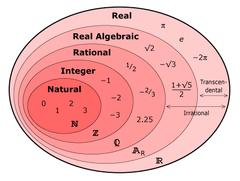positive real number
Any number that can be used to describe a physical distance greater than zero.
negative real number
Any number that can be used to describe the negative counterpart of a positive real number.
four basic math operations
Each number in an addition problem.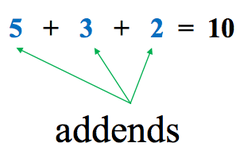sum
The result of an addition problem.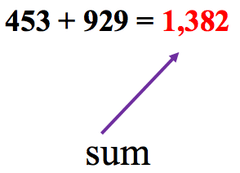difference
The result of subtraction problem.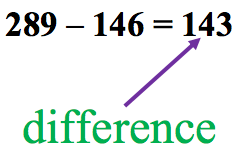factor
The numbers in a multiplication problem.product
The result of a multiplication problem.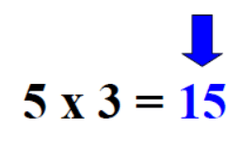quotient
The result of a division problem.fraction
A division problem represent as such: 3/4.
unit multipliers
Fractions used to change the units of a measurement.
set
Designates a well-defined collection of numbers.
absolute value
In reference to a number, the positive number that describes the distance on a number line of the graph of the number from the origin.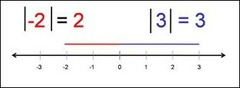If a and b are real numbers, then a - b = a +(-b)
opposite of a number
on a number line, a number that is the same distance away from zero in the other directionequation
an algebraic statement consisting of two expressions connected with an = signconstant
a quantity whose value does not change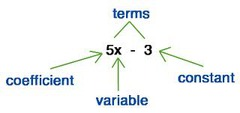equality
two expressions that are equal connected by an = signinequality
Two or more expressions connected by ≠ < or >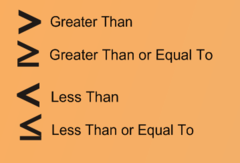real numbers
inclusive set of all numbers (counting, whole, rational & irrational)counting numbers
{ 1, 2, 3, 4, ....} also called natural numbers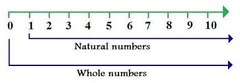whole numbers
{ 0, 1, 2, 3, 4....}integers
Positive and negative whole numbers {..., -2, -1, 0, 1, 2, 3, ....}rational numbers
number expressible as a ratio of whole numbers(can include fractions, decimals and square roots with finite results)irrational numbers
number not expressible as a ration of whole numbers(includes: π, square root of 2, etc...)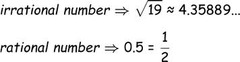Commutative Property
addition: a + b = b + a multiplication: a x b = b x a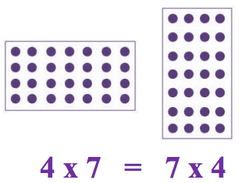inverse operations
opposite operations: addition/subtraction multiplication/divisionevery real number has an opposite that when combines makes it equal to 0 (2.56 . . . . -2.56)multiplicative inverse
every real number has a reciprocal that when combined makes it equal to 1 (3 . . . 1/3)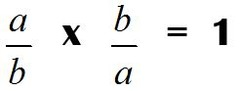term
a single entity that represents or has the value of a number {4 x xy xy/p}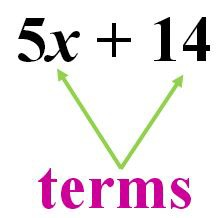coefficient
a number (numerical ) or letter (literal) which proceeds other factors in an expression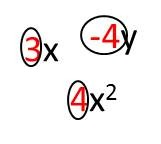factoring
writing sums as the product of factors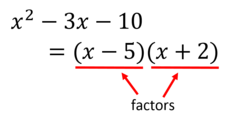Rules for terms
•numerical coefficients of like terms can be combinedRules for Equations
•additive property of equality (+/- both sides) •multiplicative property of equality (x/÷ both sides) •common factors cancel
Greatest Common Factor (GCF)
product of all prime factors common to each term, to the highest power that it occurs in all of the terms. (used for factoring)Steps: Factor to prime/use each similar factor least number of timesleast common multiple (LCM)
smallest number that can be divided evenly by each of a group of numbers (used for common denominators)Steps: Factor to prime/use each factor greatest number of times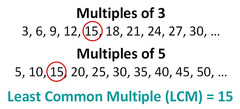equations that are second degree polynomial equations.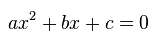polynomial
expression of more than 2 algebraic terms with different powers of the same variable An expression that can have constants (like 4) variables (like x or y) and exponents (like the 2 in y2) that can be combined using addition, subtraction, multiplication and division.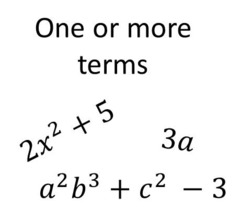Steps for Factoring
•GCF •Trinomial (foil method) •Difference of perfect squares: a²−b² factors to (a+b)(a−b) •Difference of cubes: b³-8 = (b−2)(b²+2b+4) •Perfect square: x²−6x+9 = (x−3)²Exponent
a mathematical notation indicating the number of times a quantity is multiplied by itself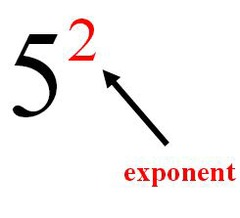power
a mathematical notation indicating the number of times a quantity is multiplied by itselfa symbol which represents the square root of a number.prime factor
Breaking down a composite number until all of the factors are primeMonomial
an expression with only one termbinomial
a polynomial with two terms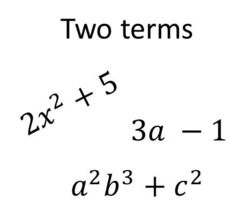Trinomial
A polynomial with three terms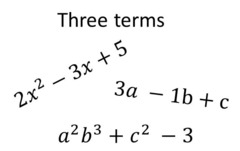Slope Intercept
y = mx + b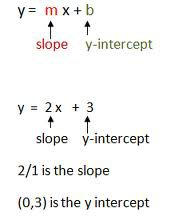consecutive integers
integers in counting order
Ratio
shows a relationship between two things,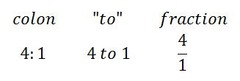proportion
A statement that two ratios are equal. To solve one, cross multiply.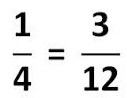33 items en en

31 items en en

28 items en en

38 items en en

22 items en en

62 items en en

56 items en en

37 items en en

29 items en en

20 items en en

23 items en en

53 items en en

45 items en en

40 items en en

25 items en en